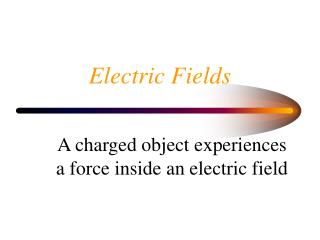DownloadDownload PresentationElectric Fields

# Electric Fields

Download Presentation## Electric Fields

- - - - - - - - - - - - - - - - - - - - - - - - - - - E N D - - - - - - - - - - - - - - - - - - - - - - - - - - -
##### Presentation Transcript

1. Electric Fields A charged object experiences a force inside an electric field

2. Electric Fields • The field does work on charged objects • Charges accelerate • W = Q x V

3. Potential Difference • Work done to move 1 coulomb of charge • 1V = 1 J C-1

4. Internal Resistance Internal resistance, r E.m.f. ( E ) Current, I E = I.R + I.r Load, R

5. Internal Resistance • Resistance of power supply itself • work is done to push charges through power supply hence ‘Lost Volts’ • E.M.F. Maximum energy to push unit charge around circuit • Terminal potential difference , work to push unit charge through external circuit ( load )

6. Internal Resistance • E = V t.p.d. + V lost • V t.p.d. = E - V lost V t.p.d = E - I x r V t.p.d E.M.F open circuit p.d. NO lost volts - slope = r I Short circuit current E = V lost

7. Wheatstone Bridge R2 R1 R3 R3 R4

8. Wheatstone Bridge • At balance point For out of balance bridge V r

9. Alternating Current Peak Voltage r.m.s. voltage

10. Alternating Current Resistance is independent of frequency

11. Capacitors • Dielectric 0 V + 5V Charge builds up on plates ( does not flow through dielectric ) 1 F = 1 C V-1

12. Capacitors • Work is done charging up Capacitor • Energy ( charge) is stored in Capacitor • Ee = 0.5.Q.V Q V Area under graph = Energy Stored Slope = Capacitance

13. Capacitors • Capacitors block d.c. yet pass a.c • Capacitors supply time delays • Capacitors used to smooth a.c. • Capacitors used as microphones

14. Capacitors • I directly proportional to frequency I f

15. Op Amps • Voltage Amplifier • Ideal Op Amp a) Input current = 0 A • b) p.d. across inverting and non inverting pin = 0V

16. Op Amps : Inverting Mode Rf Rin Vin Vout

17. Op Aps : Inverting Mode • Saturation occurs at c.a. 85 % of p.supply voltage Vout Vin

18. Op Amps : Summing Amp 2 inputs can be added together

19. Op Amps :Differential Mode • Difference between two inputs is amplified Rf R4 Rin Vout R3 V1 V2

20. Op Amps :Differential Mode • Can be connected to a wheatstone bridge • Used in ECG to subtract the 50 Hz mains hum from the heart signal

21. Op Amps : Output • Output current is of micro amp order • To drive a speaker or motor a power amp must be used I.e. an NPN transistor or a MOSFET transistor • This increases the current

22. Op Amps : Output NPN is switched on by output from op amp

23. MOSFET is switched by output from op amp